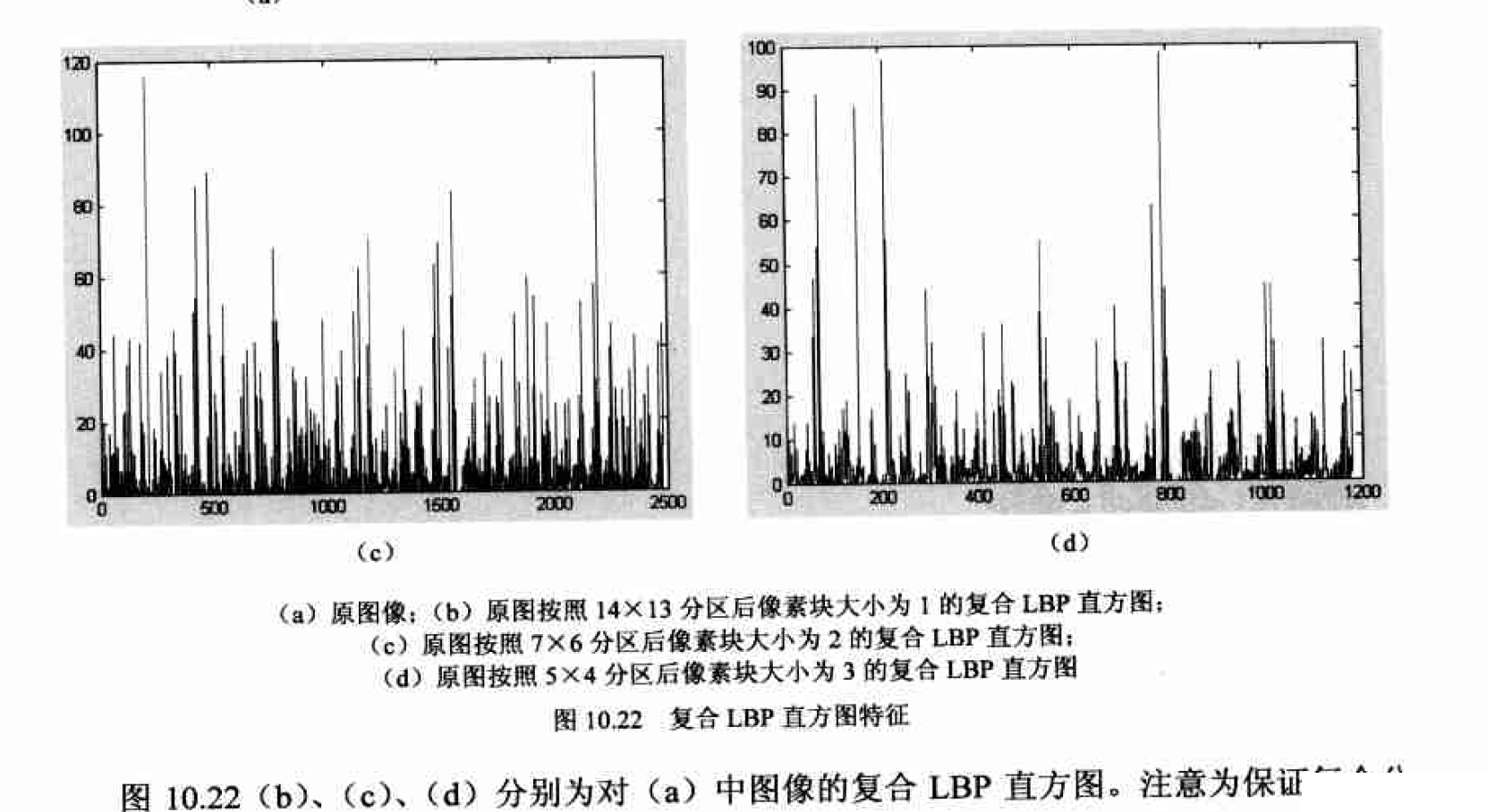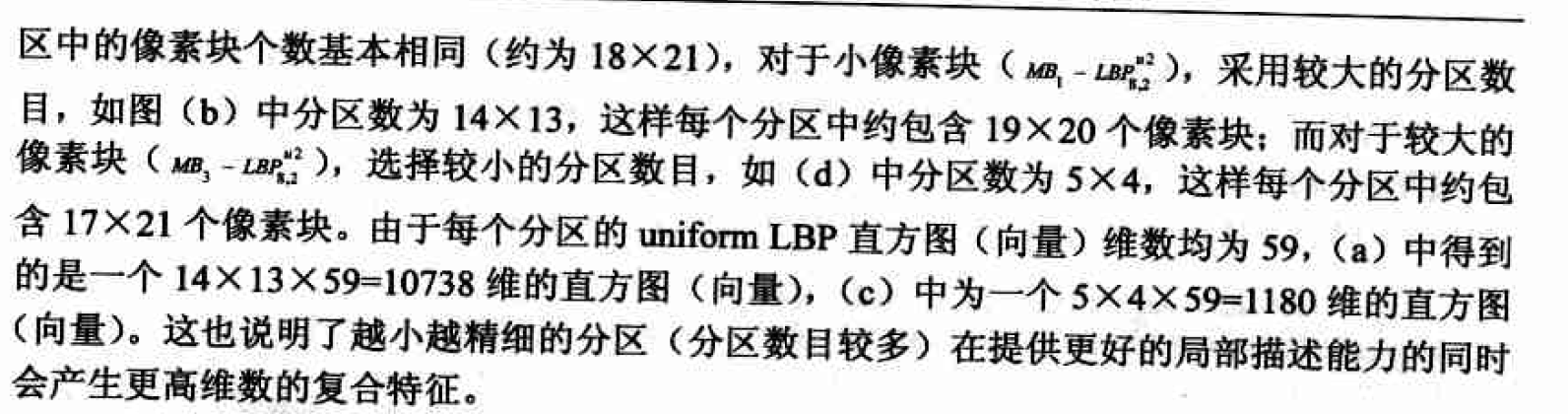2019-03-04 11:07:18 xiaoheizi_du 阅读数 3233
• ###### MATLAB图像处理

全面系统的学习MATLAB在图像处理中的应用

20258 人正在学习 去看看 魏伟

# OpenCV数字图像处理之ROI区域的提取

## 2、使用的函数简述

### (1) cv.cvtColor(img, cv.COLOR_BGR2HSV)函数

img为要进行色彩空间转换的原图
cv.COLOR_BGR2HSV即将原图RGB色彩空间转换为HSV色彩空间

### (2) cv.inRange(hsv, (h_min, s_min, v_min), (h_max, s_max, v_max))函数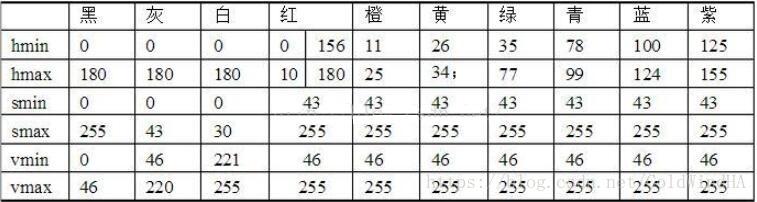## 3、代码实现过程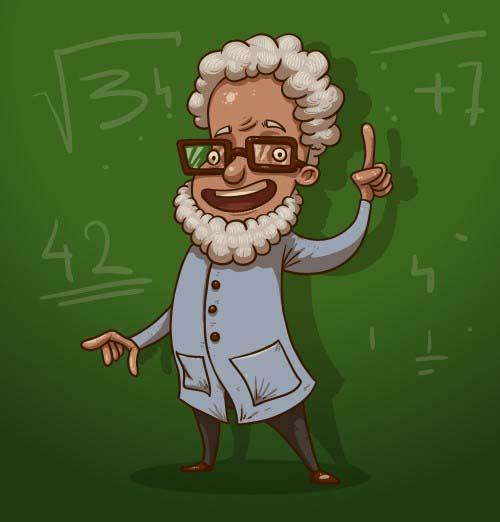### (1)读入原始图像

src = cv.imread('person.jpg')
cv.imshow('src', src)hsv = cv.cvtColor(src, cv.COLOR_BGR2HSV)       # 转换成hsv色彩风格mask = cv.bitwise_not(mask)### (4)获取人物

timg1 = cv.bitwise_and(src, src, mask=mask)
cv.imshow('timg1', timg1)### (5)新建一张与原始图一样大小的蓝色的背景图

background = np.zeros(src.shape, src.dtype)
background[:,:,0] = 255


mask = cv.bitwise_not(mask)
cv.imshow('dst1', dst)### (7)将人物图贴到蓝色背景上

dst = cv.add(dst, timg1)
cv.imshow('dst2', dst)## 4、整体代码

import cv2 as cv
import numpy as np

cv.imshow('src', src)
hsv = cv.cvtColor(src, cv.COLOR_BGR2HSV)       # 转换成hsv色彩风格

cv.imshow('timg1', timg1)
cv.imwrite('timg1.jpg', timg1)

# 生成背景
background = np.zeros(src.shape, src.dtype)
background[:,:,0] = 255

# 将人物贴到背景中
cv.imshow('dst1', dst)
cv.imwrite('dst1.jpg', dst)

cv.imshow('dst2', dst)
cv.imwrite('dst2.jpg', dst)

cv.waitKey(0)
cv.destroyAllWindows()

##### 参考

OpenCV学习笔记——HSV颜色空间超极详解&inRange函数用法及实战

2017-06-15 17:26:12 qq_22625309 阅读数 1723
• ###### MATLAB图像处理

全面系统的学习MATLAB在图像处理中的应用

20258 人正在学习 去看看 魏伟

int main()
{
Rect ROI(10, 20, 300, 400);
Mat img = image(ROI);
imwrite("result/ROI.bmp", img);
int aaa;
cin >> aaa;
return 0;
}int up = image.rows, left = image.cols, right = 0, down = 0;
int flag = 0;
int nr = image.rows, nc = image.cols;
if (image.isContinuous())
{
nr = 1;
nc = nc*image.rows;//convert 2D to 1D if the image is continuous
flag = 1;
}
//cout << "flag=" << flag << endl;
if (flag == 1)//situation 1:the image is continuous
{
for (int i = 0; i < nr; i++)
{
const uchar* inData = region.ptr<uchar>(i);
for (int j = 0; j < nc; j++)
{
if (*inData == 0 && *(inData + 1) == 255)
{
int row = (j + 1) / (region.cols);
int col = (j + 1) - row*region.cols;
if (row < up)
{
up = row;
}
if (col < left)
{
left = col;
}
}
if (*inData == 255 && *(inData + 1) == 0)
{
int row = j / (region.cols);
int col = j - row*region.cols;
if (row > down)
{
down = row;
}
if (col > right)
{
right = col;
}
}
inData++;
}
}
}
else//situation 2:the image is not continuous
{
for (int i = 0; i < nr; i++)
{
const uchar* inData = region.ptr<uchar>(i);
for (int j = 0; j < nc; j++)
{
if (*inData == 0 && *(inData + 1) == 255)
{
int row = i;
int col = j;
if (row < up)
{
up = row;
}
if (col < left)
{
left = col;
}
}
if (*inData == 255 && *(inData + 1) == 0)
{
int row = i;
int col = j;
if (row > down)
{
down = row;
}
if (col > right)
{
right = col;
}
}
inData++;
}
}
}
bb = Point2i(left, up);
bb = Point2i(right, down);
}


Rect roi(bb.x,bb.y,bb.x-bb.x,bb.y-bb.y)2019-07-23 22:46:58 guanlily123 阅读数 2163
• ###### MATLAB图像处理

全面系统的学习MATLAB在图像处理中的应用

20258 人正在学习 去看看 魏伟

（1）使用strel函数创建指定形状对应得结构元素，形状参数选择disk，根据背景复杂程度选择合适得圆盘半径。 background=imopen（I，strel（‘disk’，15））;
（2）对原始图像和由strel函数创建得结构元素进行开运算，得到背景图像。
（3）使用imsubtract函数对原始图像和背景图像做减法运算，得到消除背景后得感兴趣区域。 I2=imsubtract（I，background）;

（1）提取原图得R、G、B分量；
（2）使用ginput函数获得所选择点得坐标；
（3）使用line函数在两个相邻选择点连线，直到终点与起点重合，连成封闭区域；
（4）使用roipoly函数选择封闭折线围成得灰度图得多边形区域，背景部分为黑，区域内为白；
（5）将原图得RGB分量分别与roipoly所得到得多边形区域图像做点乘运算，并联结各分量归一化，得到去除背景保留感兴趣区域得图像。

2019-09-13 12:45:06 Vichael_Chan 阅读数 681
• ###### MATLAB图像处理

全面系统的学习MATLAB在图像处理中的应用

20258 人正在学习 去看看 魏伟

## 二、特征概述

至今为止特征没有万能和精确的定义。特征的精确定义往往由问题或者应用类型决定。特征是一个数字图像中“有趣”的部分，它是许多计算机图像分析算法的起点。因此一个算法是否成功往往由它使用和定义的特征决定。因此特征提取最重要的一个特性是“可重复性”：同一场景的不同图像所提取的特征应该是相同的。


# 三、常用图像特征概述

1.1基本概念

1.2常用的特征提取与匹配方法
（1） 颜色直方图

（2） 颜色集

（3） 颜色矩

（4） 颜色聚合向量

2.1基本概念

2.2常用的特征提取与匹配方法
（1）统计方法

（2）几何法

（3）模型法

3.1基本概念

3.2常用的特征提取与匹配方法

（1）边界特征法

（2）傅里叶形状描述符法

（3）几何参数法

（4）形状不变矩法

### 4．空间关系特征

4.1基本概念

4.2常用的特征提取与匹配方法

2017-01-03 16:38:12 whuhan2013 阅读数 88268
• ###### MATLAB图像处理

全面系统的学习MATLAB在图像处理中的应用

20258 人正在学习 去看看 魏伟

• 常用的基本统计特征， 如周长、面积、均值等区域描绘子， 以及直方图和灰度共现矩阵等纹理描绘子
• 主成份分析(PCA, PrincipaJ Component Analysis)
• 局部二进制模式(LBP, LocaJ Binary Pattern)
• 本章的典型案例分析
• 基于PCA技术的人脸数据集的降维处理

### 图像特征概述

• 特征应当容易提取． 换言之， 为了得到这些特征我们付出的代价不能太大． 当然， 这还要与特征的分类能力权衡考虑．
• 选取的特征应对噪声和不相关转换不敏感． 比如要识别车牌号码， 车牌照片可能是从各个角度拍摄的， 而我们关心的是车牌上字母和数字的内容， 因此就需要得到对几何失真变形等转换不敏感的描绘子， 从而得到旋转不变， 或是投影失真不变的特征．
• 最重要的一点， 总是应试图寻找最具区分能力的特征．

### 基本统计特征

#### 简单的区域描绘子及其Matlab实现

• 周长：区域边界的长度， 即位于区域边界上的像素数目．
• 面积：， 区域中的像素总数．
• 致密性：（周长） 2/面积．
• 区域的质心．
• 灰度均值： 区域中所有像素的平均值．
• 灰度中值： 区域中所有像素的排序中值．
• 包含区域的最小矩形．
• 最小或最大灰度级．
• 大于或小于均值的像素数．
• 欧拉数： 区域中的对象数减去这些对象的孔洞数。

D = regionprops(L,properties)
L是一个标记矩阵， 可通过8.3.4小节介绍的连通区标注函数bwlabel得到．
properties可以是一个用逗号分割的字符串列表， 其一些常用取值如表10.1所示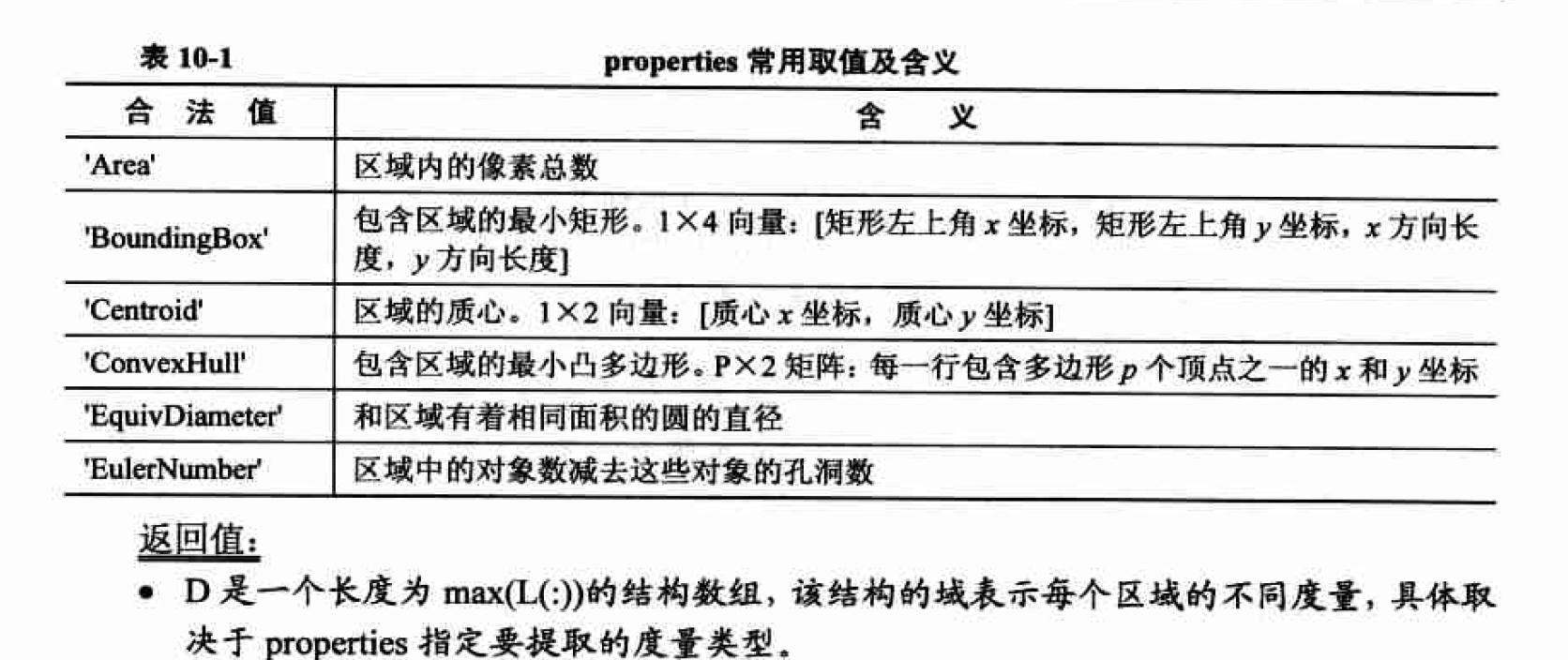I = imread('bw_mouth.bmp');
I1 = bwlabel(I);
D = regionprops(I1,'area','centroid');
D.Area

#### 直方图及其统计特征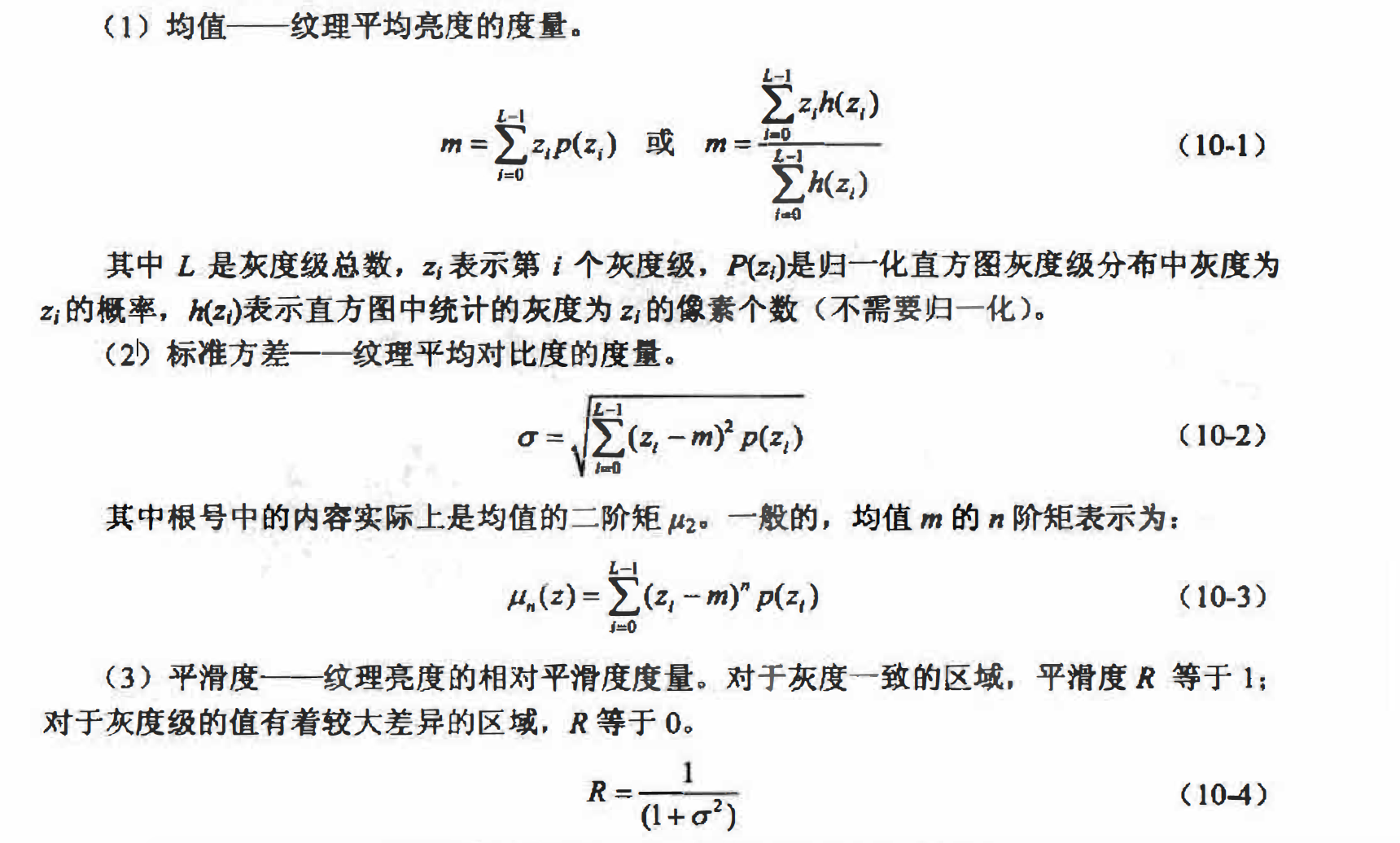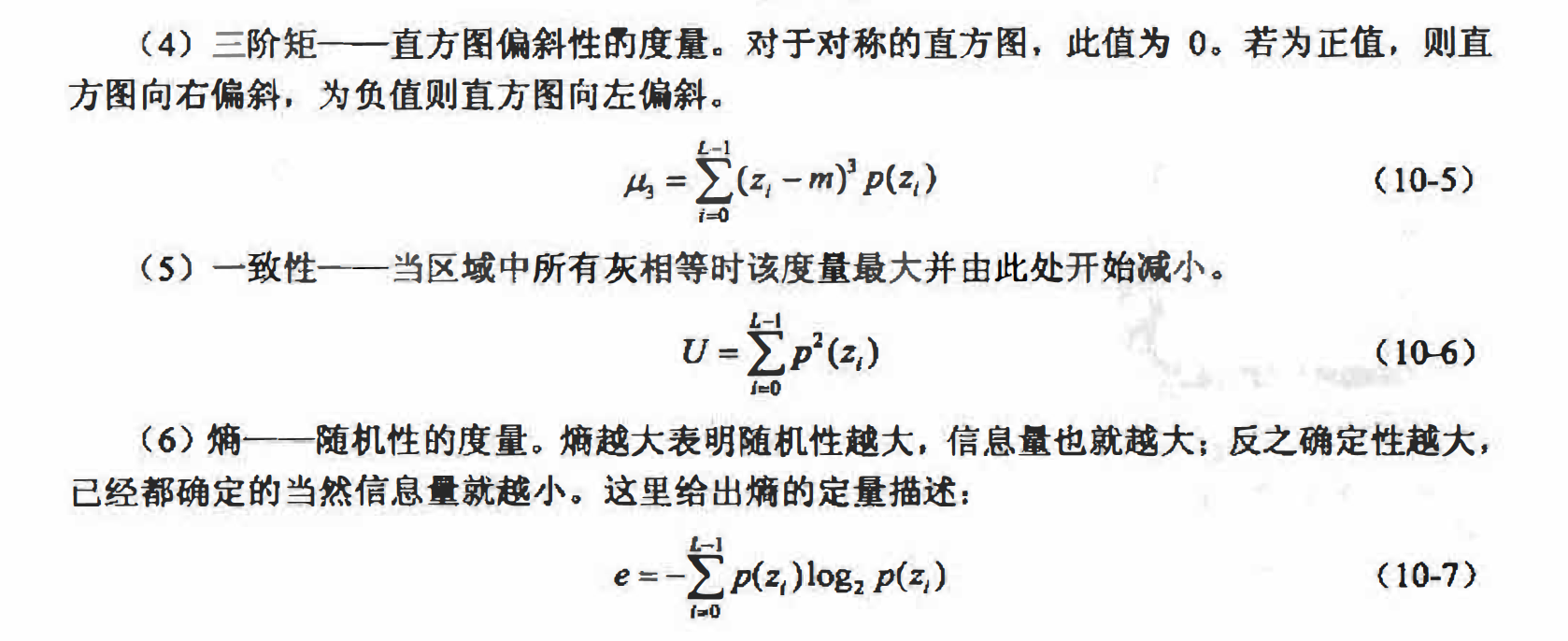### 灰度共现矩阵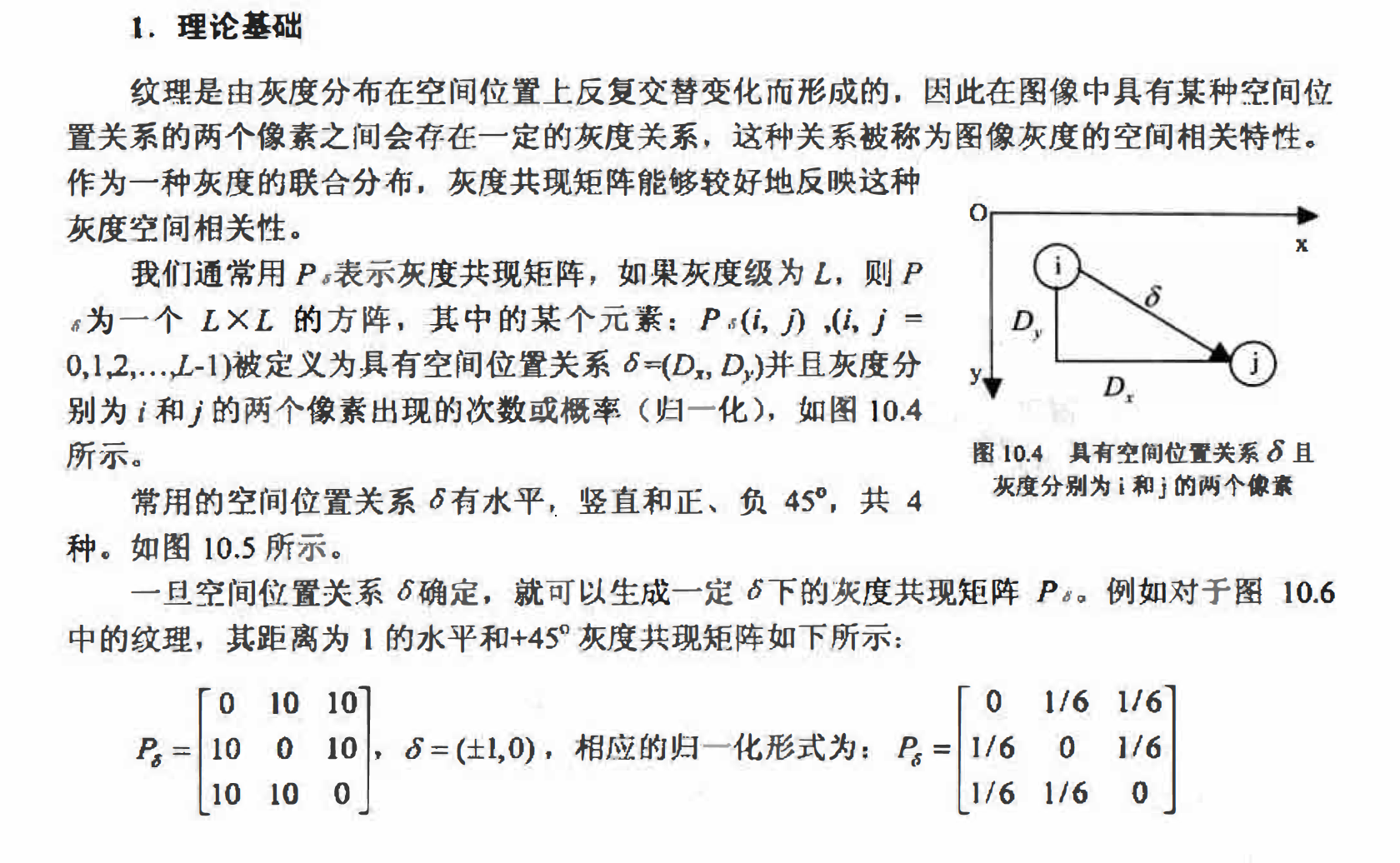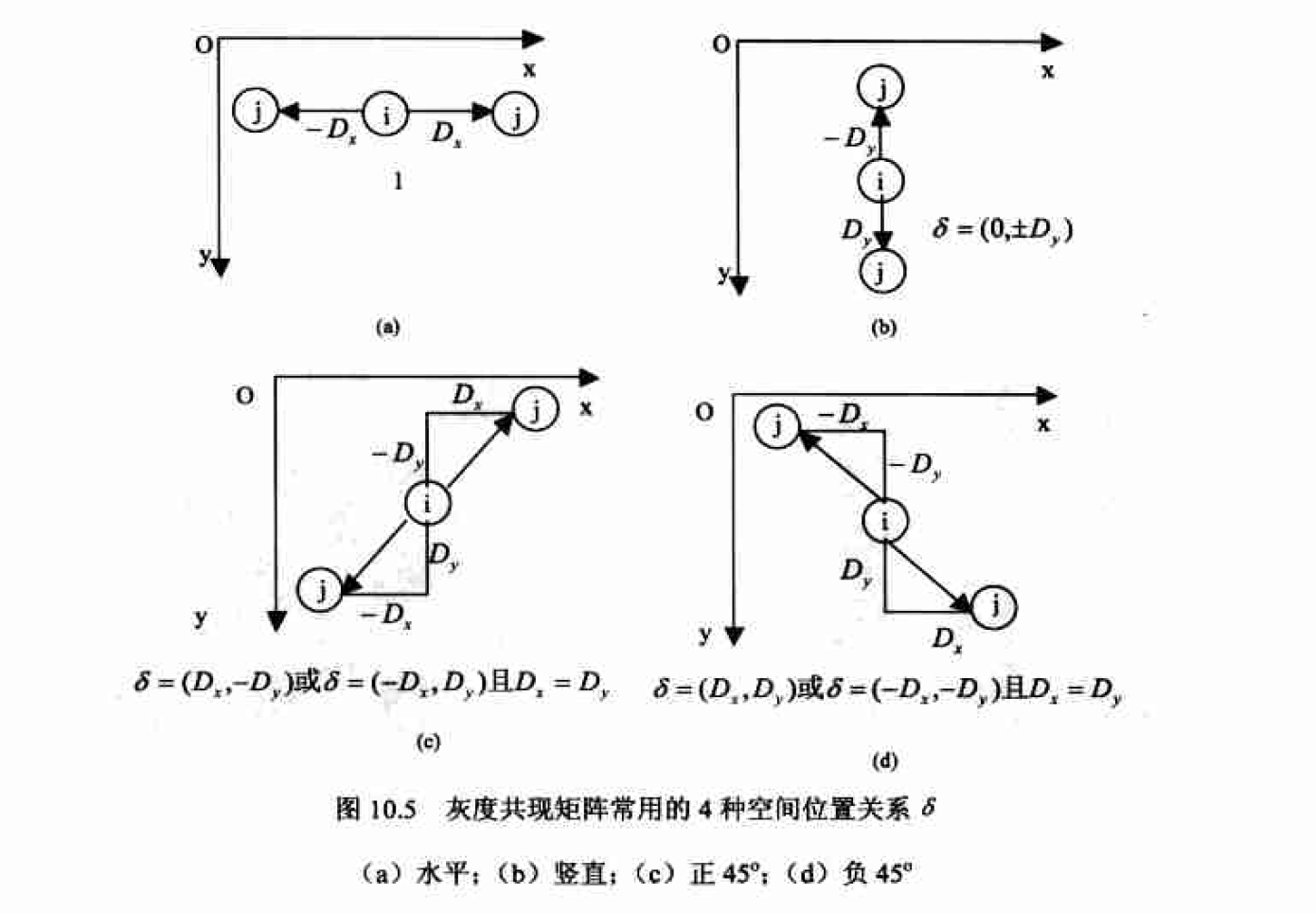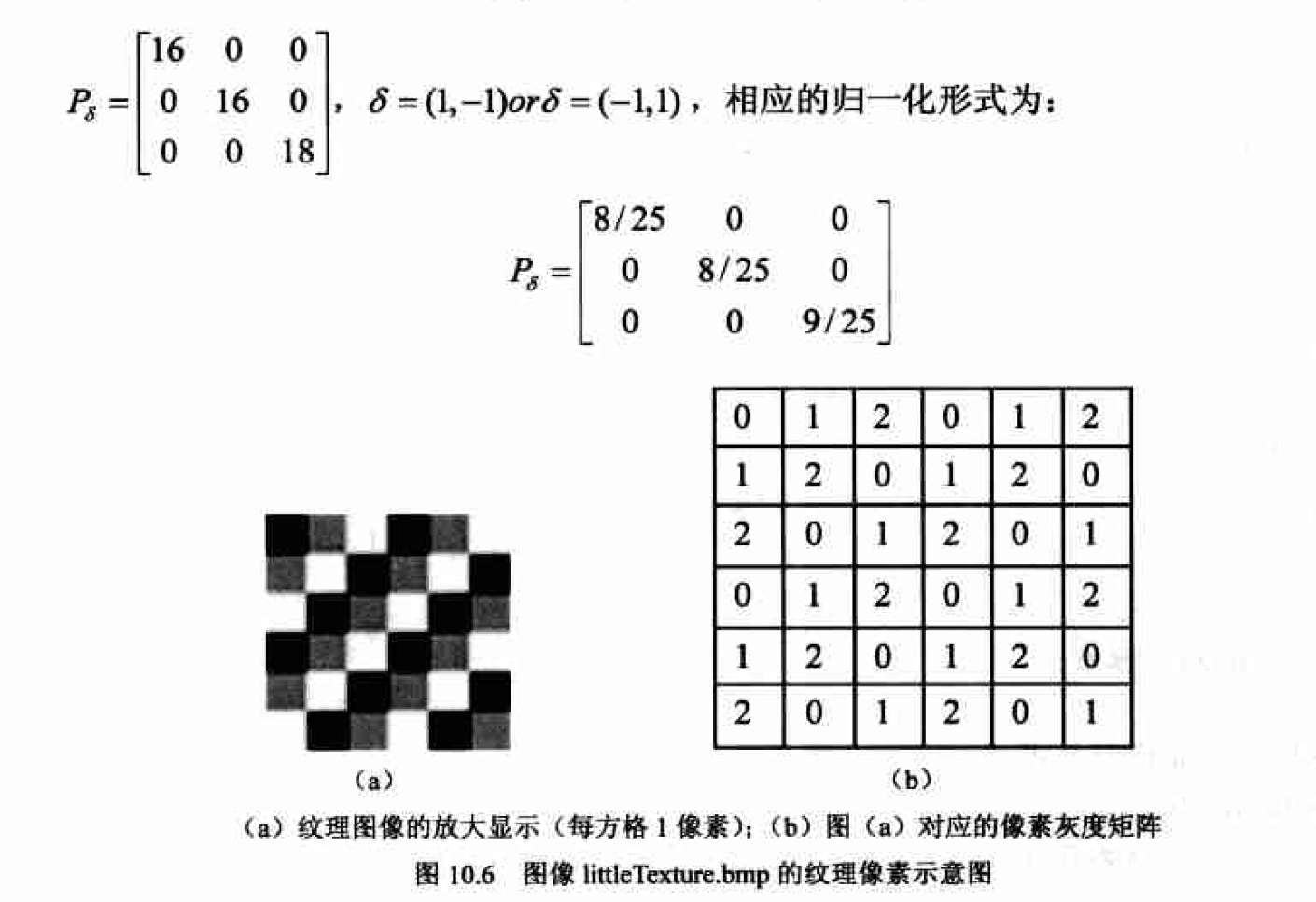### 特征降维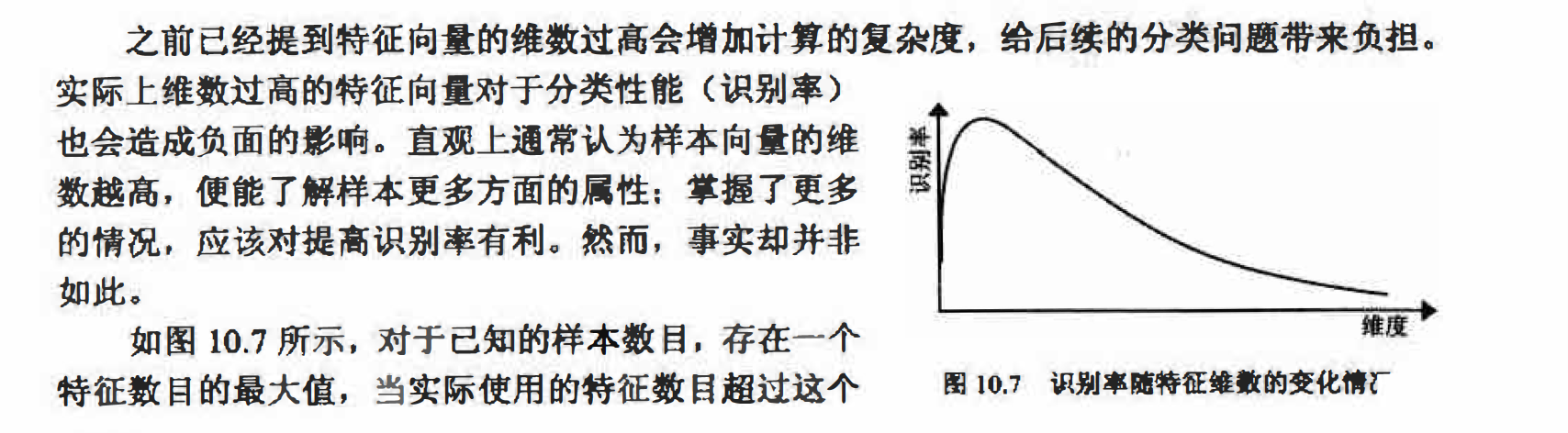#### 特征选择简介

load fisheriris;
data = [meas(:,1),meas(:,2)];
figure;
scatter(data(1:50,1),data(1:50,2),'b+');
hold on,scatter(data(51:100,1),data(51:100,2),'r*');
hold on,scatter(data(101:150,1),data(101:150,2),'go');

data = [meas(:,1),meas(:,3)];
figure;
scatter(data(1:50,1),data(1:50,2),'b+');
hold on,scatter(data(51:100,1),data(51:100,2),'r*');
hold on,scatter(data(101:150,1),data(101:150,2),'go');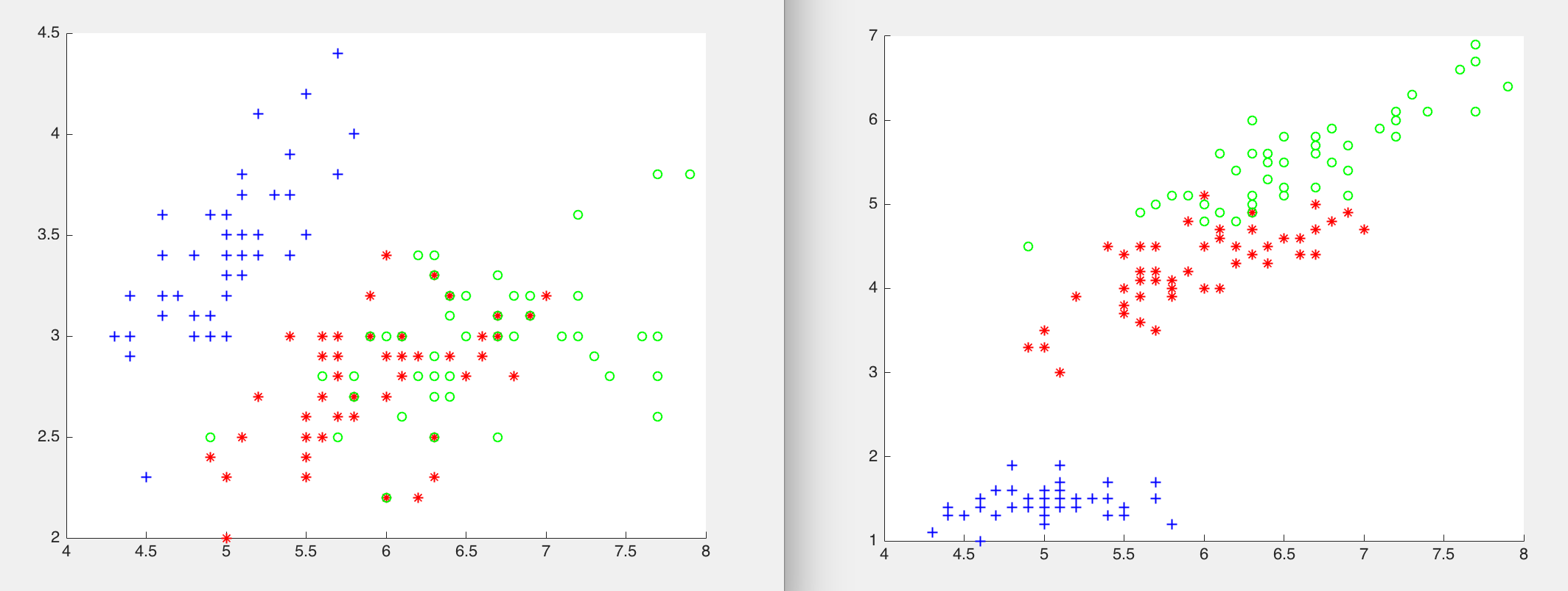#### 主成分分析(Princjpal Component Analysis, PCA)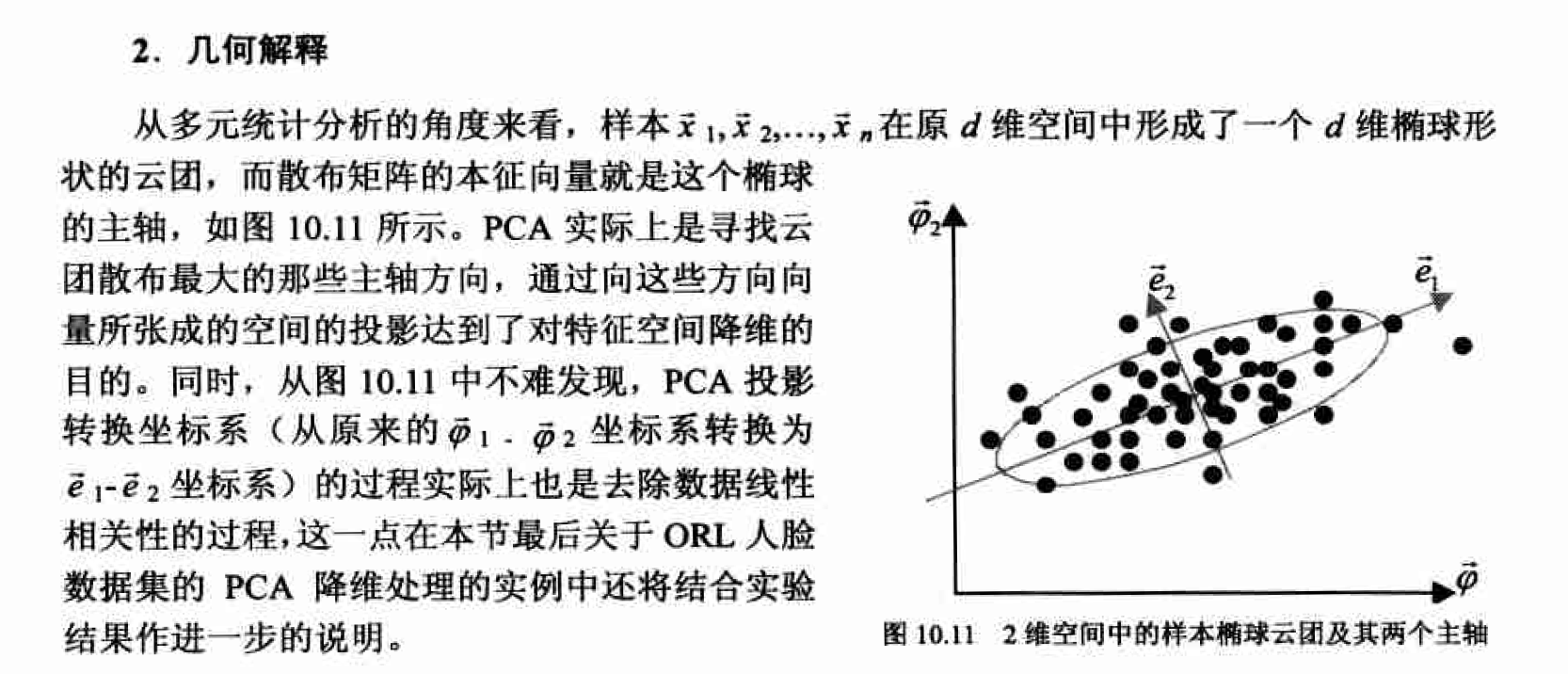PCA计算实例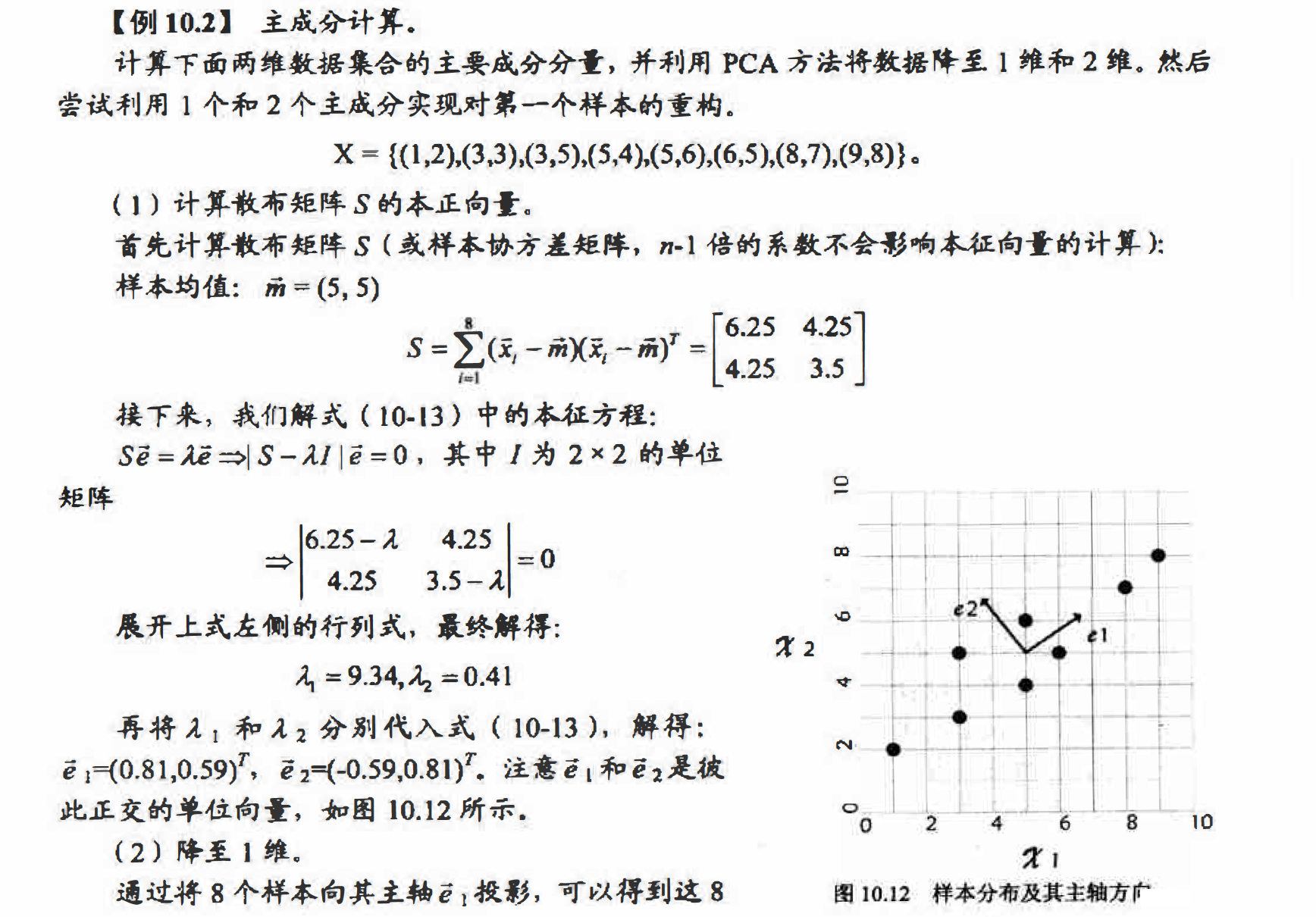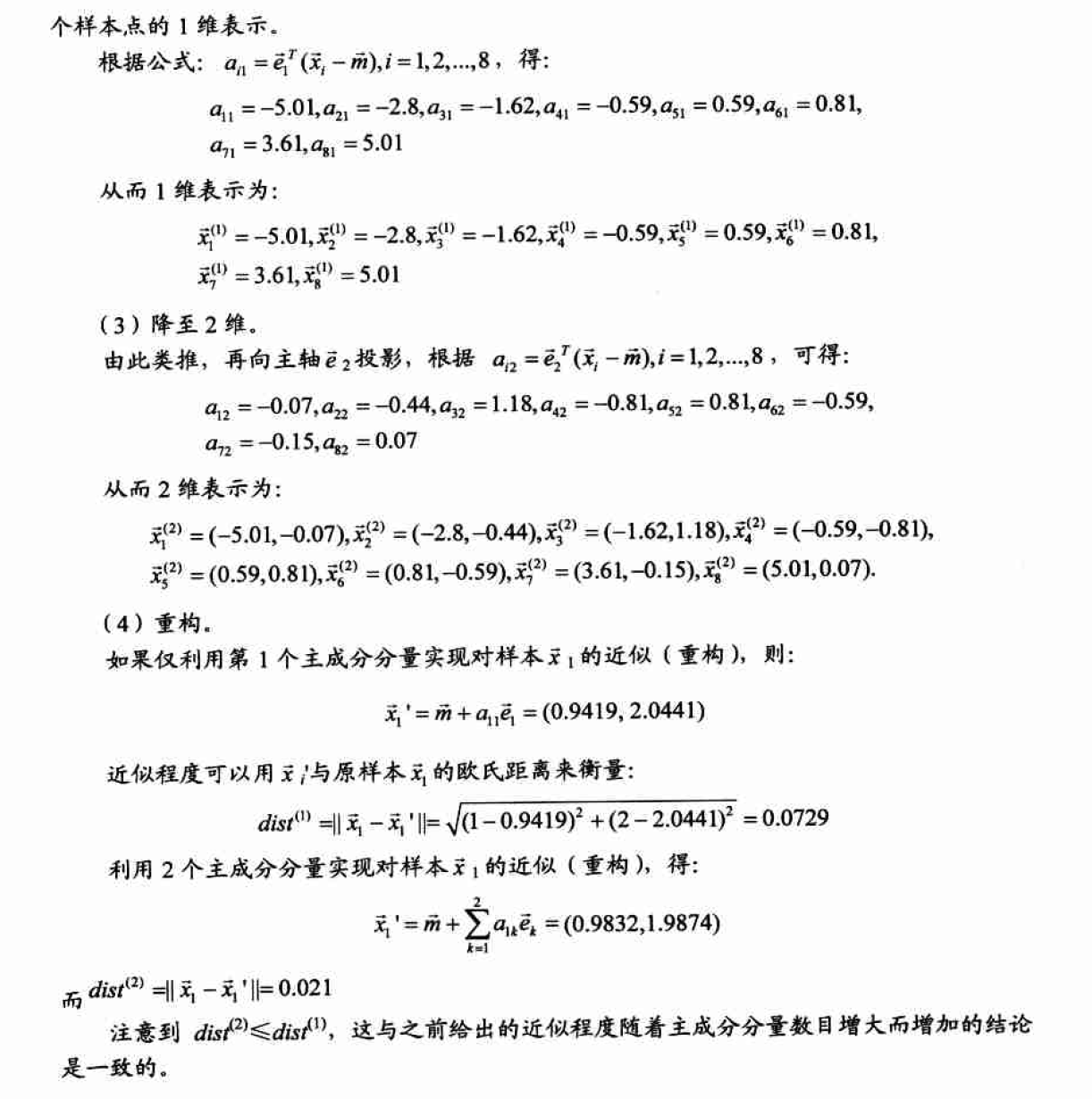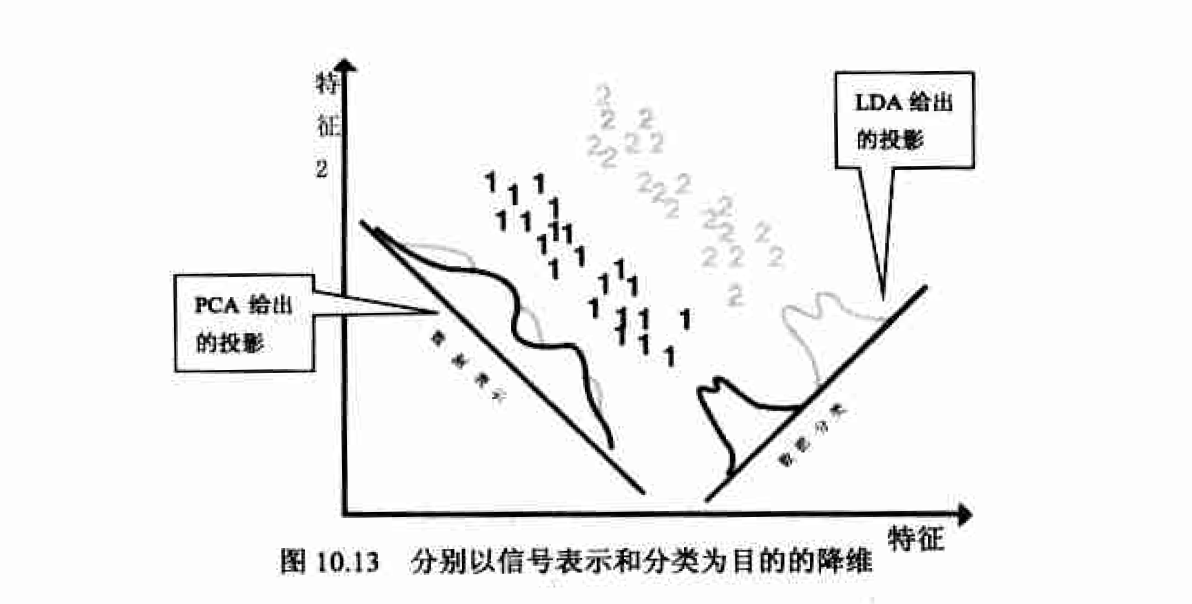PCA的Matlab实现

[COEFF,SCORE,latent]= princomp(X);
X为原始样本组成n*d的矩阵，其每一行是一个样本特征向量，每一列表示样本特征向量的一维．如对于例10.2中的问题，X就是一个8*2的样本矩阵， 总共8个样本， 每个样本2维．
COEFF: 主成份分量， 即变换空间中的那些基向量, 也是样本协方差矩阵的本征向量．
SCORE: 主成份，X的低维表示， 即X中的数据在主成分分量上的投影（可根据需要取前面几列的).
latent: 一个包含着样本协方差矩阵本征值的向量．

PCA的计算中最主要的工作量是计算样本协方差矩阵的本征值和本征向量。设样本矩阵 X大小为n*d (n个d维样本特征向量）， 则样本散布矩阵（协方差矩阵） S将是一个dXd的方阵，故当维数d较大时计算复杂度会非常高。例如当维数d=1OOOO,S是一个10000*10000的矩阵，此时如果采用上面的princomp函数计算主成分，Matlab通常会出现内存耗尽的错误，即使有足够多的内存， 要得到S的全部本征值可能也要花费数小时的时间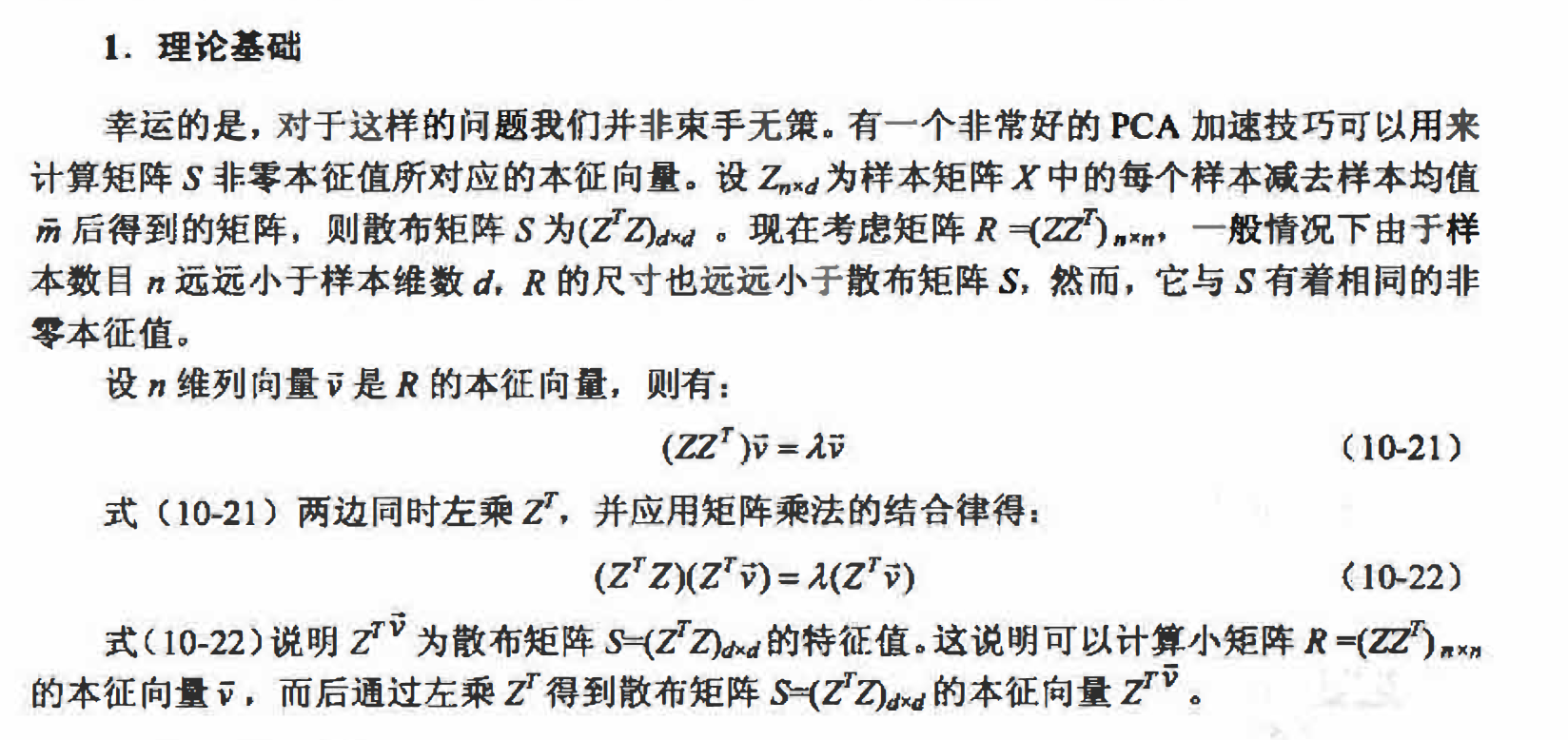Matlab实现

function [pcaA V] = fastPCA( A, k )
% 快速PCA
%
% 输入：A --- 样本矩阵，每行为一个样本
%      k --- 降维至 k 维
%
% 输出：pcaA --- 降维后的 k 维样本特征向量组成的矩阵，每行一个样本，列数 k 为降维后的样本特征维数
%      V --- 主成分向量

[r c] = size(A);

% 样本均值
meanVec = mean(A);

% 计算协方差矩阵的转置 covMatT
Z = (A-repmat(meanVec, r, 1));
covMatT = Z * Z';

% 计算 covMatT 的前 k 个本征值和本征向量
[V D] = eigs(covMatT, k);

% 得到协方差矩阵 (covMatT)' 的本征向量
V = Z' * V;

% 本征向量归一化为单位本征向量
for i=1:k
V(:,i)=V(:,i)/norm(V(:,i));
end

% 线性变换（投影）降维至 k 维
pcaA = Z * V;

% 保存变换矩阵 V 和变换原点 meanVec
save('Mat/PCA.mat', 'V', 'meanVec');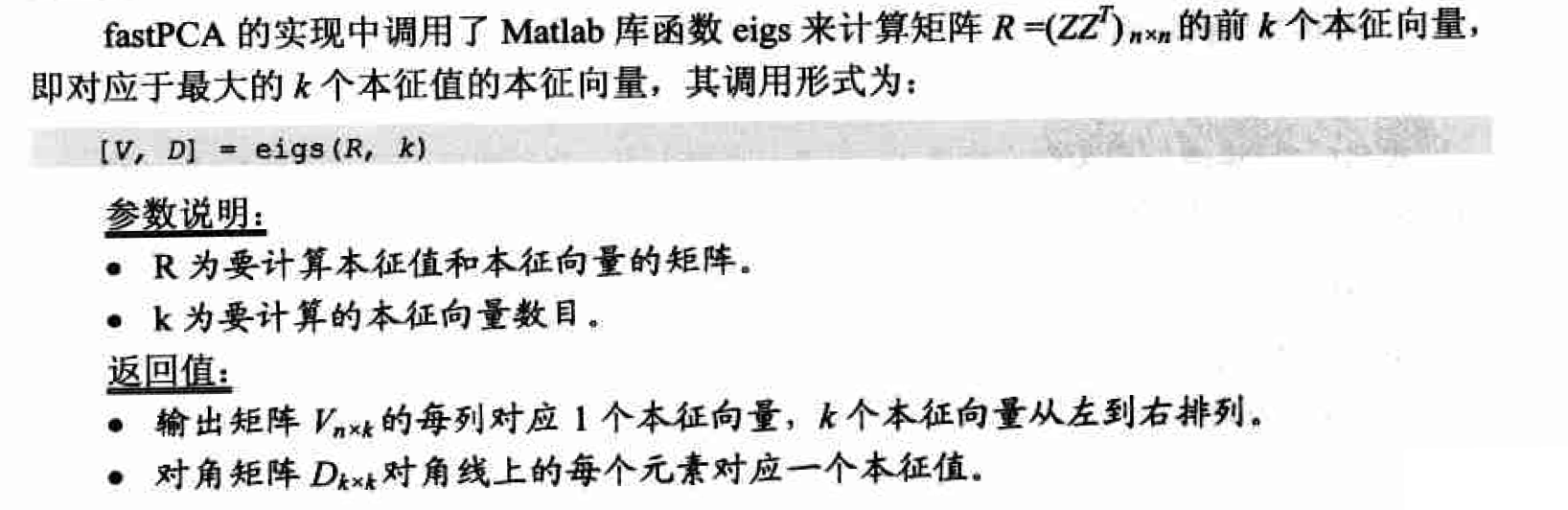### 局部二进制模式

#### 基本LBP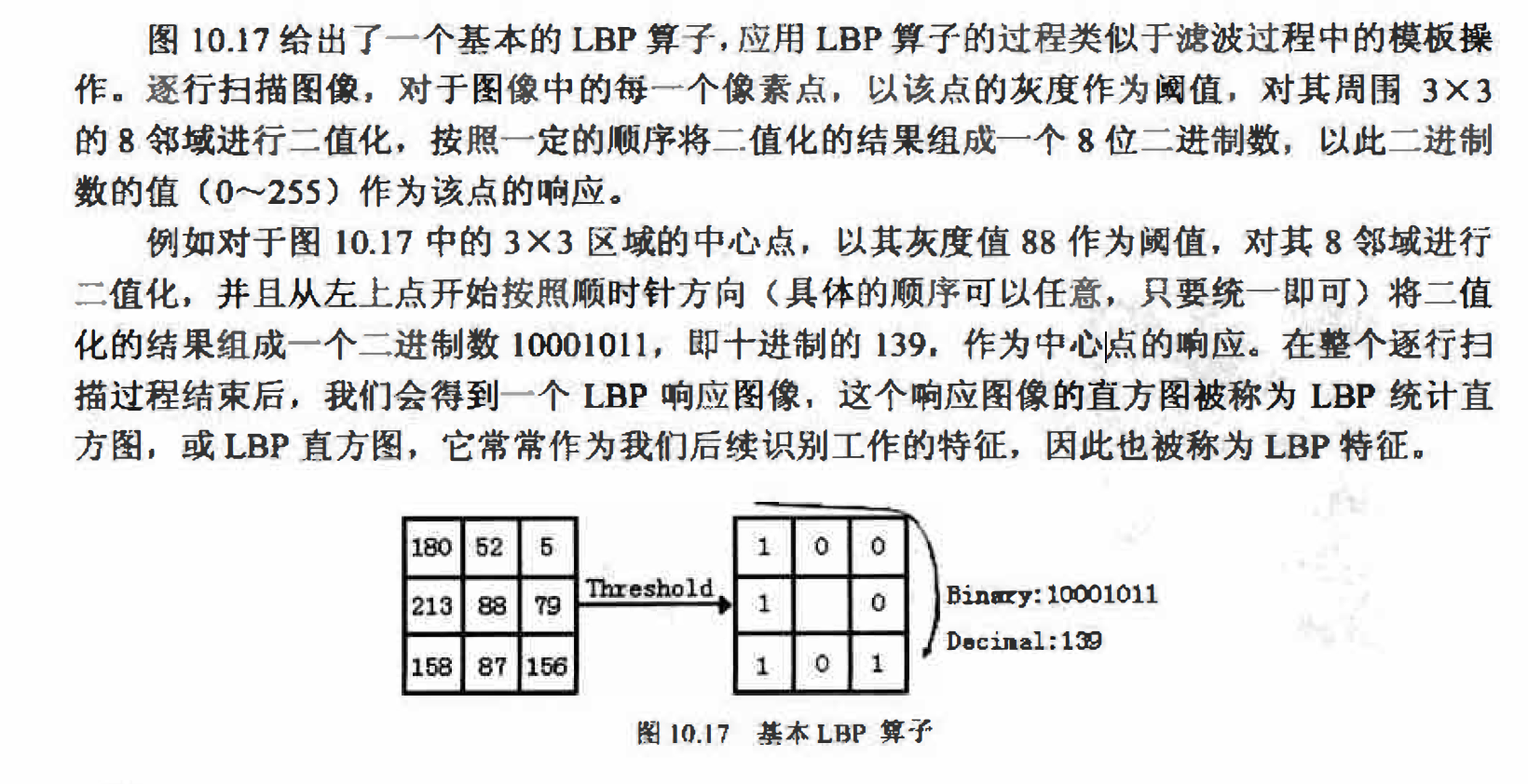LBP的主要思想是以某一点与其邻域像素的相对灰度作为响应， 正是这种相对机制使 LBP算子对于单调的灰度变化具有不变性。 人脸图像常常会受到光照因素的影响而产生灰度变化，但在一个局部区域内，这种变化常常可以被视为是单调的，因此LBP在光照不均的人 脸识别应用中也取得了很好的效果.

#### 圆形邻域的LBPP,R$LBP_{P,R}$算子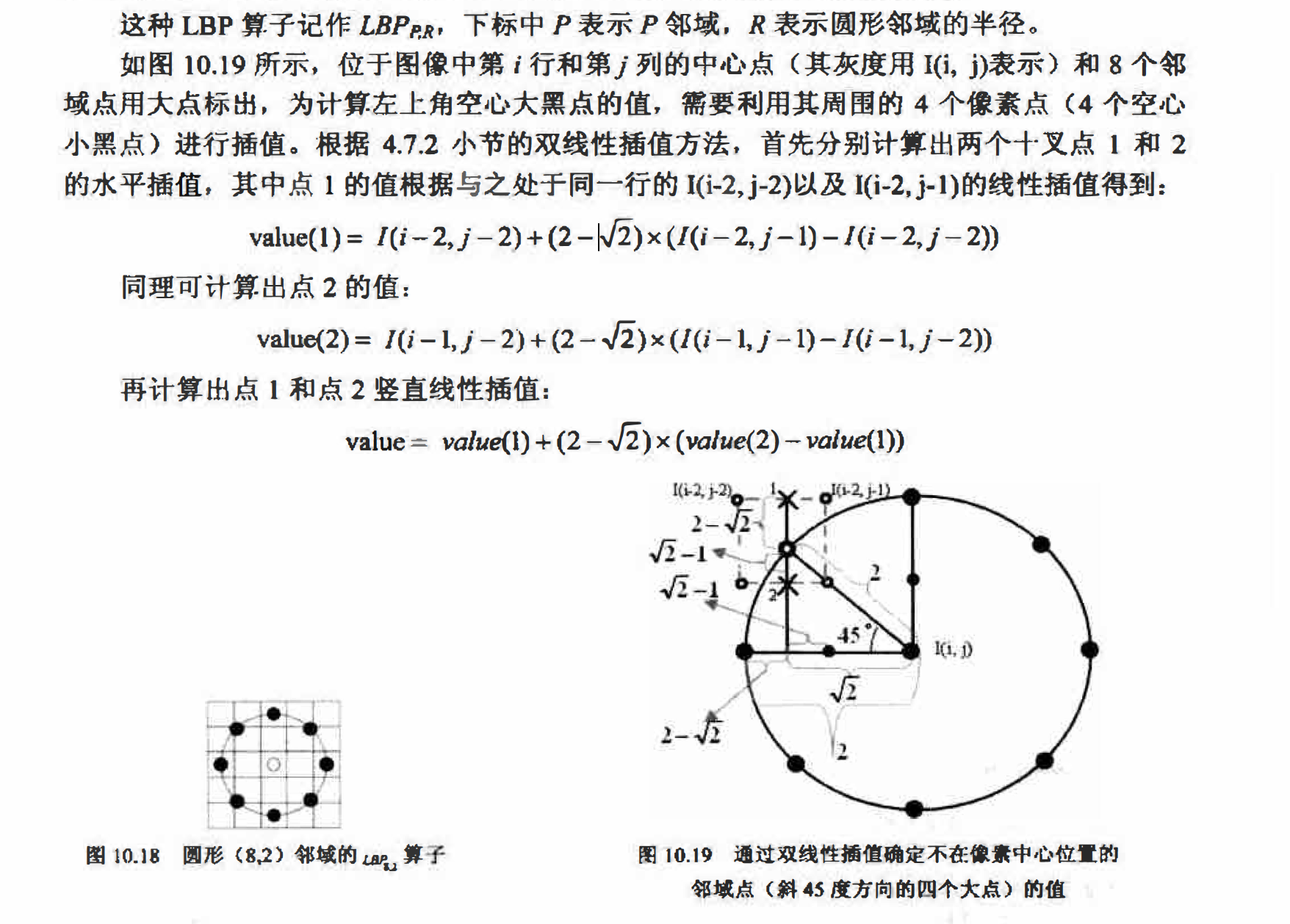#### 统一化LBP算子一一UnifomLBP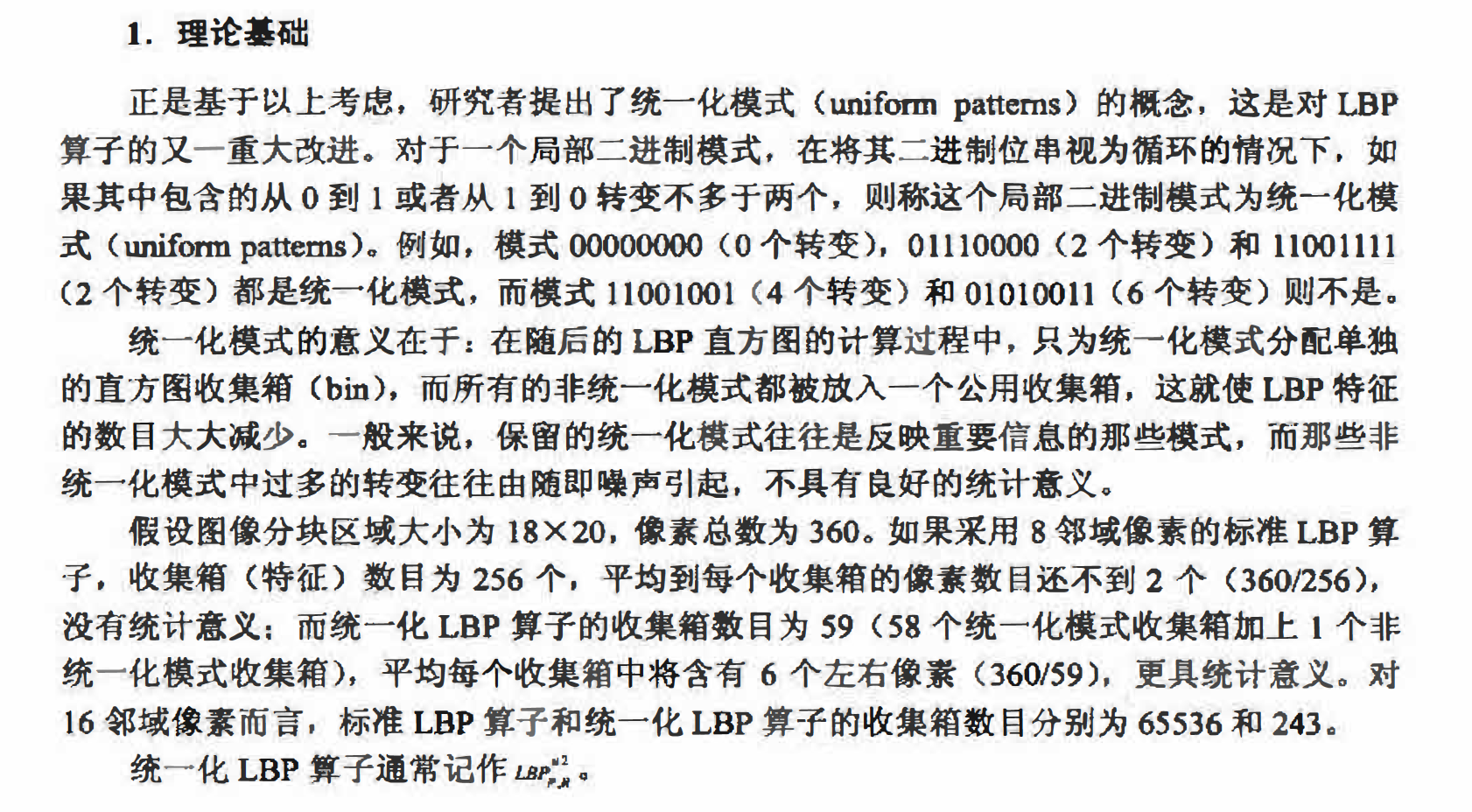#### MB-LBP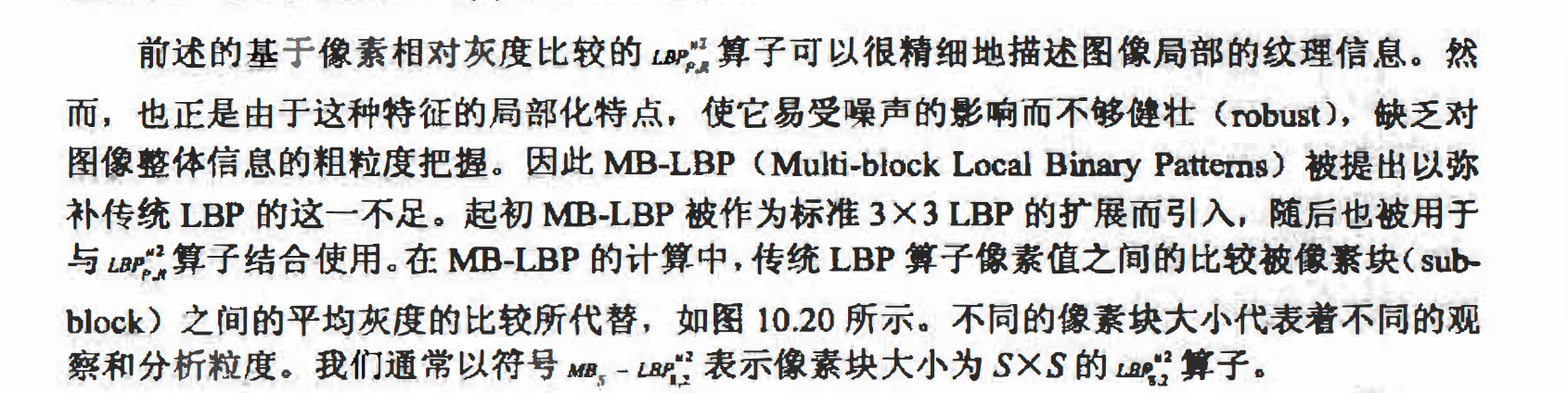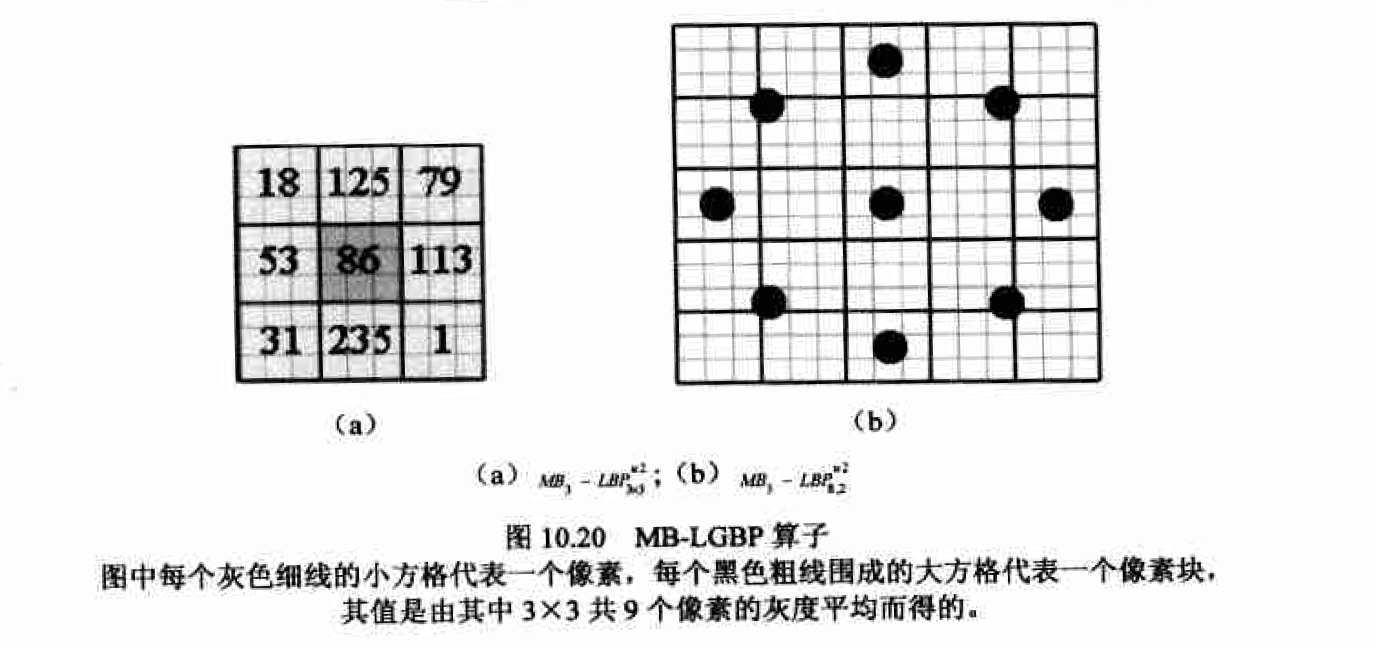#### 图像分区

feature)作为代表整个图像的LBP直方图特征。